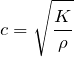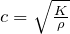# Speed of Sound in Liquid

Speed of Sound in a Liquid (Static Fluid Wave Speed)

Summary

In a fluid at rest, the wave speed of a fluid is equivalent to the speed of sound in the same medium, whether liquid or gas.

The speed of sound is calculated from the Newton-Laplace equation:

(1)Where c = speed of sound, K = bulk modulus or stiffness coefficient, ρ = density.

Where a pressure wave passes through a liquid contained within an elastic vessel, the liquid’s density and therefore the wave speed will change as the pressure wave passes. This is commonly experienced as water hammer.

Speed of Sound in Static Liquid Calculator

 Calculate speed of sound in liquid: Formula:c = speed of sound K = bulk modulus ρ = density Bulk modulus: Density: kg/m³ Result: m/s